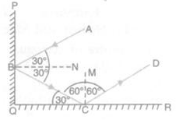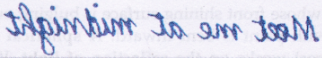# Solutions of Reflection of Light (Page No- 175) - Physics By Lakhmir Singh, Class 10 Class 10 Notes | EduRev

## Class 10 : Solutions of Reflection of Light (Page No- 175) - Physics By Lakhmir Singh, Class 10 Class 10 Notes | EduRev

The document Solutions of Reflection of Light (Page No- 175) - Physics By Lakhmir Singh, Class 10 Class 10 Notes | EduRev is a part of the Class 10 Course Class 10 Physics Solutions By Lakhmir Singh & Manjit Kaur.
All you need of Class 10 at this link: Class 10

Lakhmir Singh Physics Class 10 Solutions Page No:175

Question 36: A man stands 10 m in front of a large plane mirror. How far must he walk before he is 5 m away from his image ?

Solution : Initially, the distance between the man and the mirror is 10m.
So, the distance between man and his image is 10+10 = 20m
Distance between the man and his image is 5 m when the man is 2.5 m away from the mirror.
Therefore, he has to walk 10 m – 2.5m = 7.5 m towards the mirror

Question 37: An object is placed 20 cm in front of a plane mirror. The mirror is moved 2 cm towards the object. The distance between the positions of the original and final images seen in the mirror is :
(a) 2 cm,
(b) 4 cm
(c) 10 cm
(d) 22 cm

Solution : Initially, the object is placed 20 cm in front of mirror. So the image will form 20 cm behind the mirror.
If mirror is moved 2 cm towards the object,
distance between object and mirror = 20 cm – 2 cm = 18 cm
distance between mirror and image = 18 cm, which is 2 cm less than the initial case.
Since, the mirror has also moved 2 cm away from the position of original image, so the total distance between the positions of the original and final images is 2 cm + 2 cm = 4 cm

Question 38: A man sits in an optician’s chair, looking into a plane mirror which is 2 m away from him and views the image of a chart which faces the mirror and is 50 cm behind his head. How far away from his eyes does the chart appear to be ?

Solution : Distance between the man and the mirror = 2 cm
Distance between man and chart = 50 cm = 0.5 m
Distance between chart and mirror = 0.5 m + 2 m = 2.5 m
Distance between mirror and the image of the chart = 2.5 m
Distance between man and the image of chart = Distance between man and the mirror + Distance between mirror and the image of the chart = 2 m + 2.5 m = 4.5 m

Question 39: A ray of light strikes a plane mirror PQ at an angle of incidence of 30°, is reflected from the plane mirror and then strikes a second plane mirror QR placed at right angles to the first mirror. The angle of reflection at the second mirror is :
(a) 30° (b) 45° (c) 60° (d) 90°

Solution :Ray AB strikes the mirror PQ at B and gets reflected along BC according to the laws of reflection. The ray BC incident on mirror QR makes an angle of 30o with the mirror. So, the angle of incidence on this mirror is 90o-30= 60o. Hence, the angle of reflection is also 60o.

Question 40: Explain how to read the following message which was found on some blotting paper :Solution : The impression on blotting paper is the mirror image of the written massage. Therefore, to read this message we need to hold the written message in front of a mirror so that the mirror produces a laterally inverted image of this message

Offer running on EduRev: Apply code STAYHOME200 to get INR 200 off on our premium plan EduRev Infinity!

94 docs

,

,

,

,

,

,

,

,

,

,

,

,

,

,

,

,

,

,

,

,

,

,

,

,

;